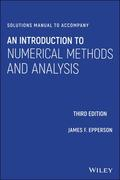John Wiley & Sons Solutions Manual to accompany An Introduction to Numerical Methods and AnalysisA solutions manual to accompany An Introduction to Numerical Methods and Analysis, Third Edition An.. Product #: 978-1-119-60453-2 Regular price: \$27.94 \$27.94 In Stock

# Solutions Manual to accompany An Introduction to Numerical Methods and Analysis3. Edition September 2021
304 Pages, Softcover
Wiley & Sons Ltd

ISBN: 978-1-119-60453-2
John Wiley & Sons

### Price: 29,90 €

Price incl. VAT, excl. Shipping

A solutions manual to accompany An Introduction to Numerical Methods and Analysis, Third Edition

An Introduction to Numerical Methods and Analysis helps students gain a solid understanding of a wide range of numerical approximation methods for solving problems of mathematical analysis. Designed for entry-level courses on the subject, this popular textbook maximizes teaching flexibility by first covering basic topics before gradually moving to more advanced material in each chapter and section. Throughout the text, students are provided clear and accessible guidance on a wide range of numerical methods and analysis techniques, including root-finding, numerical integration, interpolation, solution of systems of equations, and many others.

This fully revised third edition contains new sections on higher-order difference methods, the bisection and inertia method for computing eigenvalues of a symmetric matrix, a completely re-written section on different methods for Poisson equations, and spectral methods for higher-dimensional problems. New problem sets--ranging in difficulty from simple computations to challenging derivations and proofs--are complemented by computer programming exercises, illustrative examples, and sample code. This acclaimed textbook:
* Explains how to both construct and evaluate approximations for accuracy and performance
* Covers both elementary concepts and tools and higher-level methods and solutions
* Features new and updated material reflecting new trends and applications in the field
* Contains an introduction to key concepts, a calculus review, an updated primer on computer arithmetic, a brief history of scientific computing, a survey of computer languages and software, and a revised literature review
* Includes an appendix of proofs of selected theorems and author-hosted companion website with additional exercises, application models, and supplemental resources

1 Introductory Concepts and Calculus Review 1

1.1 Basic Tools of Calculus 1

1.2 Error, Approximate Equality, and Asymptotic Order Notation 10

1.3 A Primer on Computer Arithmetic 13

1.4 A Word on Computer Languages and Software 17

1.5 A Brief History of Scientific Computing 18

2 A Survey of Simple Methods and Tools 19

2.1 Horner's Rule and Nested Multiplication 19

2.2 Difference Approximations to the Derivative 22

2.3 Application: Euler's Method for Initial Value Problems 30

2.4 Linear Interpolation 34

2.5 Application--The Trapezoid Rule 38

2.6 Solution of Tridiagonal Linear Systems 46

2.7 Application: Simple Two-Point Boundary Value Problems 50

3 Root-Finding 55

3.1 The Bisection Method 55

3.2 Newton's Method: Derivation and Examples 59

3.3 How to Stop Newton's Method 63

3.4 Application: Division Using Newton's Method 66

3.5 The Newton Error Formula 69

3.6 Newton's Method: Theory and Convergence 72

3.7 Application: Computation of the Square Root 76

3.8 The Secant Method: Derivation and Examples 79

3.9 Fixed Point Iteration 83

3.10 Roots of Polynomials (Part 1) 85

3.11 Special Topics in Root-finding Methods 88

3.12 Very High-order Methods and the Efficiency Index 98

4 Interpolation and Approximation 101

4.1 Lagrange Interpolation 101

4.2 Newton Interpolation and Divided Differences 104

4.3 Interpolation Error 114

4.4 Application: Muller's Method and Inverse Quadratic Interpolation 119

4.5 Application: More Approximations to the Derivative 121

4.6 Hermite Interpolation 122

4.7 Piecewise Polynomial Interpolation 125

4.8 An Introduction to Splines 129

4.9 Tension Splines 135

4.10 Least Squares Concepts in Approximation 137

4.11 Advanced Topics in Interpolation Error 142

5 Numerical Integration 149

5.1 A Review of the Definite Integral 149

5.2 Improving the Trapezoid Rule 151

5.3 Simpson's Rule and Degree of Precision 154

5.4 The Midpoint Rule 162

5.5 Application: Stirling's Formula 166

5.7 Extrapolation Methods 173

5.8 Special Topics in Numerical Integration 177

6 Numerical Methods for Ordinary Differential Equations 185

6.1 The Initial Value Problem--Background 185

6.2 Euler's Method 187

6.3 Analysis of Euler's Method 189

6.4 Variants of Euler's Method 190

6.5 Single Step Methods--Runge-Kutta 197

6.6 Multistep Methods 200

6.7 Stability Issues 204

6.8 Application to Systems of Equations 206

6.10 Boundary Value Problems 212

7 Numerical Methods for the Solution of Systems of Equations 217

7.1 Linear Algebra Review 217

7.2 Linear Systems and Gaussian Elimination 218

7.3 Operation Counts 223

7.4 The LU Factorization 224

7.5 Perturbation, Conditioning and Stability 229

7.6 SPD Matrices and the Cholesky Decomposition 235

7.7 Application: Numerical Solution of Linear Least Squares Problems 236

7.8 Sparse and Structured Matrices 240

7.9 Iterative Methods for Linear Systems - A Brief Survey 241

7.10 Nonlinear Systems: Newton's Method and Related Ideas 242

7.11 Application: Numerical Solution of Nonlinear BVP's 244

8 Approximate Solution of the Algebraic Eigenvalue Problem 247

8.1 Eigenvalue Review 247

8.2 Reduction to Hessenberg Form 249

8.3 Power Methods 250

8.4 Bisection and Inertia to Compute Eigenvalues of Symmetric Matrices 253

8.5 An Overview of the QR Iteration 257

8.6 Application: Roots of Polynomials, II 260

8.7 Application: Computation of Gaussian Quadrature Rules 261

9 A Survey of Numerical Methods for Partial Differential Equations 265

9.1 Difference Methods for the Diffusion Equation 265

9.2 Finite Element Methods for the Diffusion Equation 270

9.3 Difference Methods for Poisson Equations 271

10 An Introduction to Spectral Methods 277

10.1 Spectral Methods for Two-Point Boundary Value Problems 277

10.2 Spectral Methods in Two Dimensions 279

10.3 Spectral Methods for Time-Dependent Problems 282